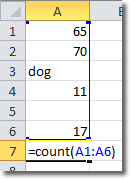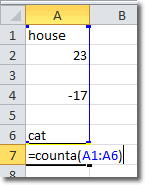# Planet For Application Life Development Presents MY IT WorldExplore and uptodate your technology skills...

Excel - Count

The Excel COUNT function counts the number of cells in a range that contain numbers. This function will not include blank cells or cells that contain non-numeric values (like words). To use the count function, type “=COUNT(A1:A10)” into the cell you want to hold the result. A1 to A10 is the range I chose, so you should substitute that with your own range.

Let’s consider the following cells in a spreadsheet:After typing =COUNT(A1:A6) and pressing Enter, Excel calculates the count to be 4.  There are 6 cells in the selected range, but one contains the word “dog” and one cell is blank. That means there are 4 cells that contain numeric values and so the count is 4.

## Count Nonblank Cells

There is another count function that you can use in Excel: COUNTA. COUNTA returns a count of all the nonblank cells in a range.

Consider the following spreadsheet:There are 6 cells in the range A1:A6. 2 are blank, 2 contain words and 2 contain numbers. This means that there are 4 nonblank cells (2 numbers and 2 words) so the COUNTA function returns 4.